Anúncio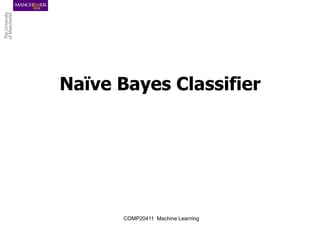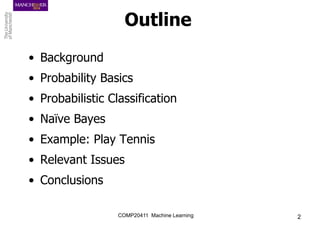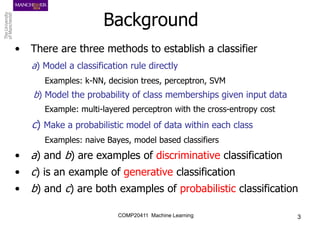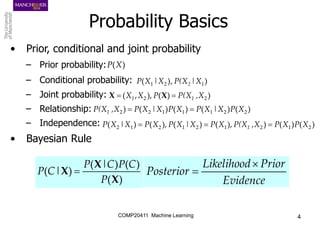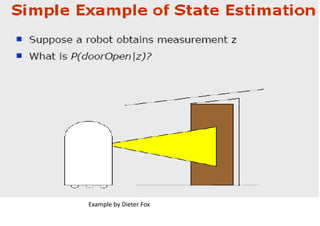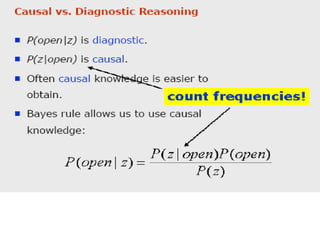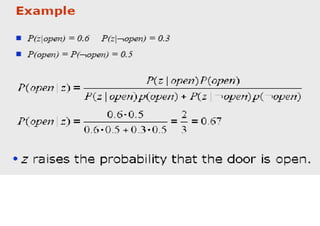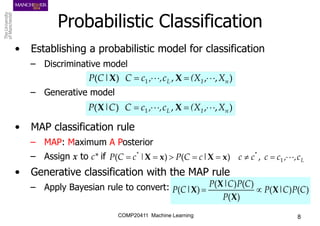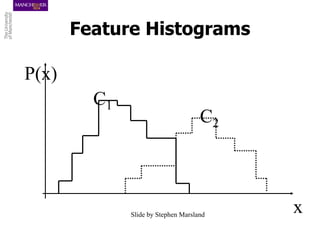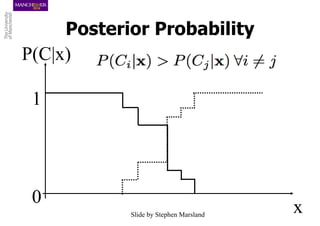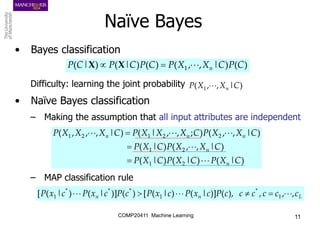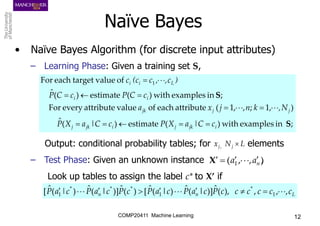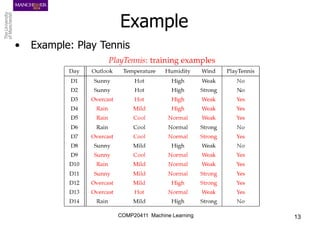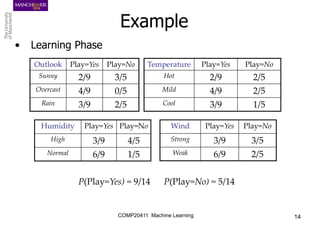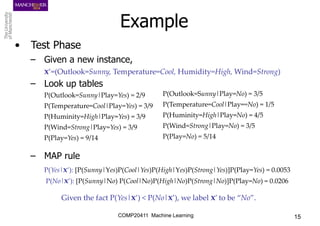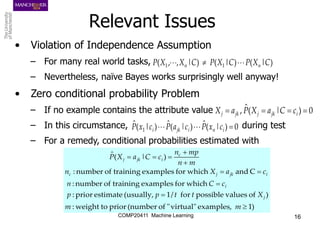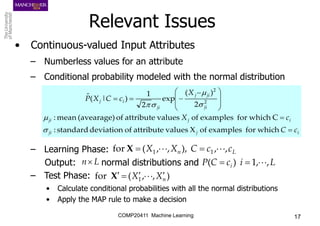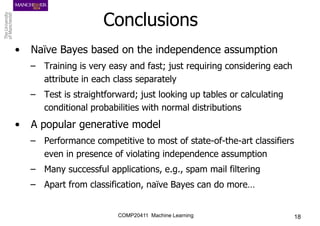1 de 18
Anúncio

### ch8Bayes.pptx

1. Naïve Bayes Classifier COMP20411 Machine Learning
2. COMP20411 Machine Learning 2 Outline • Background • Probability Basics • Probabilistic Classification • Naïve Bayes • Example: Play Tennis • Relevant Issues • Conclusions
3. COMP20411 Machine Learning 3 Background • There are three methods to establish a classifier a) Model a classification rule directly Examples: k-NN, decision trees, perceptron, SVM b) Model the probability of class memberships given input data Example: multi-layered perceptron with the cross-entropy cost c) Make a probabilistic model of data within each class Examples: naive Bayes, model based classifiers • a) and b) are examples of discriminative classification • c) is an example of generative classification • b) and c) are both examples of probabilistic classification
4. COMP20411 Machine Learning 4 Probability Basics • Prior, conditional and joint probability – Prior probability: – Conditional probability: – Joint probability: – Relationship: – Independence: • Bayesian Rule ) | , ) ( 1 2 1 X P(X X | X P 2 ) ( ) ( ) ( ) ( X X X P C P C | P | C P  ) (X P ) ) ( ), , ( 2 2 ,X P(X P X X 1 1   X X ) ( ) | ( ) ( ) | ( ) 2 2 1 1 1 2 2 X P X X P X P X X P ,X P(X1   ) ( ) ( ) ), ( ) | ( ), ( ) | ( 2 1 2 1 2 1 2 1 2 X P X P ,X P(X X P X X P X P X X P 1    Evidence Prior Likelihood Posterior  
5. Example by Dieter Fox
6. COMP20411 Machine Learning 8 Probabilistic Classification • Establishing a probabilistic model for classification – Discriminative model – Generative model • MAP classification rule – MAP: Maximum A Posterior – Assign x to c* if • Generative classification with the MAP rule – Apply Bayesian rule to convert: ) , , , ) ( 1 n 1 L X (X c , , c C | C P         X X ) , , , ) ( 1 n 1 L X (X c , , c C C | P         X X L c , , c c c c | c C P | c C P           1 * * , ) ( ) ( x X x X ) ( ) ( ) ( ) ( ) ( ) ( C P C | P P C P C | P | C P X X X X  
7. Feature Histograms x C1 C2 P(x) Slide by Stephen Marsland
8. Posterior Probability x P(C|x) 1 0 Slide by Stephen Marsland
9. COMP20411 Machine Learning 11 Naïve Bayes • Bayes classification Difficulty: learning the joint probability • Naïve Bayes classification – Making the assumption that all input attributes are independent – MAP classification rule ) ( ) | , , ( ) ( ) ( ) ( 1 C P C X X P C P C | P | C P n      X X ) | , , ( 1 C X X P n    ) | ( ) | ( ) | ( ) | , , ( ) | ( ) | , , ( ) ; , , | ( ) | , , , ( 2 1 2 1 2 2 1 2 1 C X P C X P C X P C X X P C X P C X X P C X X X P C X X X P n n n n n                   L n n c c c c c c P c x P c x P c P c x P c x P , , , ), ( )] | ( ) | ( [ ) ( )] | ( ) | ( [ 1 * 1 * * * 1            
10. COMP20411 Machine Learning 12 Naïve Bayes • Naïve Bayes Algorithm (for discrete input attributes) – Learning Phase: Given a training set S, Output: conditional probability tables; for elements – Test Phase: Given an unknown instance , Look up tables to assign the label c* to X’ if ; in examples with ) | ( estimate ) | ( ˆ ) , 1 ; , , 1 ( attribute each of value attribute every For ; in examples with ) ( estimate ) ( ˆ of value target each For 1 S S i jk j i jk j j j jk i i L i i c C a X P c C a X P N , k n j x a c C P c C P ) c , , c (c c                     L n n c c c c c c P c a P c a P c P c a P c a P , , , ), ( ˆ )] | ( ˆ ) | ( ˆ [ ) ( ˆ )] | ( ˆ ) | ( ˆ [ 1 * 1 * * * 1                 ) , , ( 1 n a a        X L N x j j  ,
11. COMP20411 Machine Learning 13 Example • Example: Play Tennis
12. COMP20411 Machine Learning 14 Example • Learning Phase Outlook Play=Yes Play=No Sunny 2/9 3/5 Overcast 4/9 0/5 Rain 3/9 2/5 Temperature Play=Yes Play=No Hot 2/9 2/5 Mild 4/9 2/5 Cool 3/9 1/5 Humidity Play=Yes Play=No High 3/9 4/5 Normal 6/9 1/5 Wind Play=Yes Play=No Strong 3/9 3/5 Weak 6/9 2/5 P(Play=Yes) = 9/14 P(Play=No) = 5/14
13. COMP20411 Machine Learning 15 Example • Test Phase – Given a new instance, x’=(Outlook=Sunny, Temperature=Cool, Humidity=High, Wind=Strong) – Look up tables – MAP rule P(Outlook=Sunny|Play=No) = 3/5 P(Temperature=Cool|Play==No) = 1/5 P(Huminity=High|Play=No) = 4/5 P(Wind=Strong|Play=No) = 3/5 P(Play=No) = 5/14 P(Outlook=Sunny|Play=Yes) = 2/9 P(Temperature=Cool|Play=Yes) = 3/9 P(Huminity=High|Play=Yes) = 3/9 P(Wind=Strong|Play=Yes) = 3/9 P(Play=Yes) = 9/14 P(Yes|x’): [P(Sunny|Yes)P(Cool|Yes)P(High|Yes)P(Strong|Yes)]P(Play=Yes) = 0.0053 P(No|x’): [P(Sunny|No) P(Cool|No)P(High|No)P(Strong|No)]P(Play=No) = 0.0206 Given the fact P(Yes|x’) < P(No|x’), we label x’ to be “No”.
14. COMP20411 Machine Learning 16 Relevant Issues • Violation of Independence Assumption – For many real world tasks, – Nevertheless, naïve Bayes works surprisingly well anyway! • Zero conditional probability Problem – If no example contains the attribute value – In this circumstance, during test – For a remedy, conditional probabilities estimated with ) | ( ) | ( ) | , , ( 1 1 C X P C X P C X X P n n        0 ) | ( ˆ ,     i jk j jk j c C a X P a X 0 ) | ( ˆ ) | ( ˆ ) | ( ˆ 1        i n i jk i c x P c a P c x P ) 1 examples, virtual" " of (number prior to weight : ) of values possible for / 1 (usually, estimate prior : which for examples training of number : C and which for examples training of number : ) | ( ˆ           m m X t t p p c C n c a X n m n mp n c C a X P j i i jk j c c i jk j
15. COMP20411 Machine Learning 17 Relevant Issues • Continuous-valued Input Attributes – Numberless values for an attribute – Conditional probability modeled with the normal distribution – Learning Phase: Output: normal distributions and – Test Phase: • Calculate conditional probabilities with all the normal distributions • Apply the MAP rule to make a decision i j ji i j ji ji ji j ji i j c C c X X c C X P               which for examples of X values attribute of deviation standard : C which for examples of values attribute of (avearage) mean : 2 ) ( exp 2 1 ) | ( ˆ 2 2       L n c c C X X , , ), , , ( for 1 1         X L n ) , , ( for 1 n X X        X L i c C P i , , 1 ) (     
16. COMP20411 Machine Learning 18 Conclusions • Naïve Bayes based on the independence assumption – Training is very easy and fast; just requiring considering each attribute in each class separately – Test is straightforward; just looking up tables or calculating conditional probabilities with normal distributions • A popular generative model – Performance competitive to most of state-of-the-art classifiers even in presence of violating independence assumption – Many successful applications, e.g., spam mail filtering – Apart from classification, naïve Bayes can do more…
Anúncio